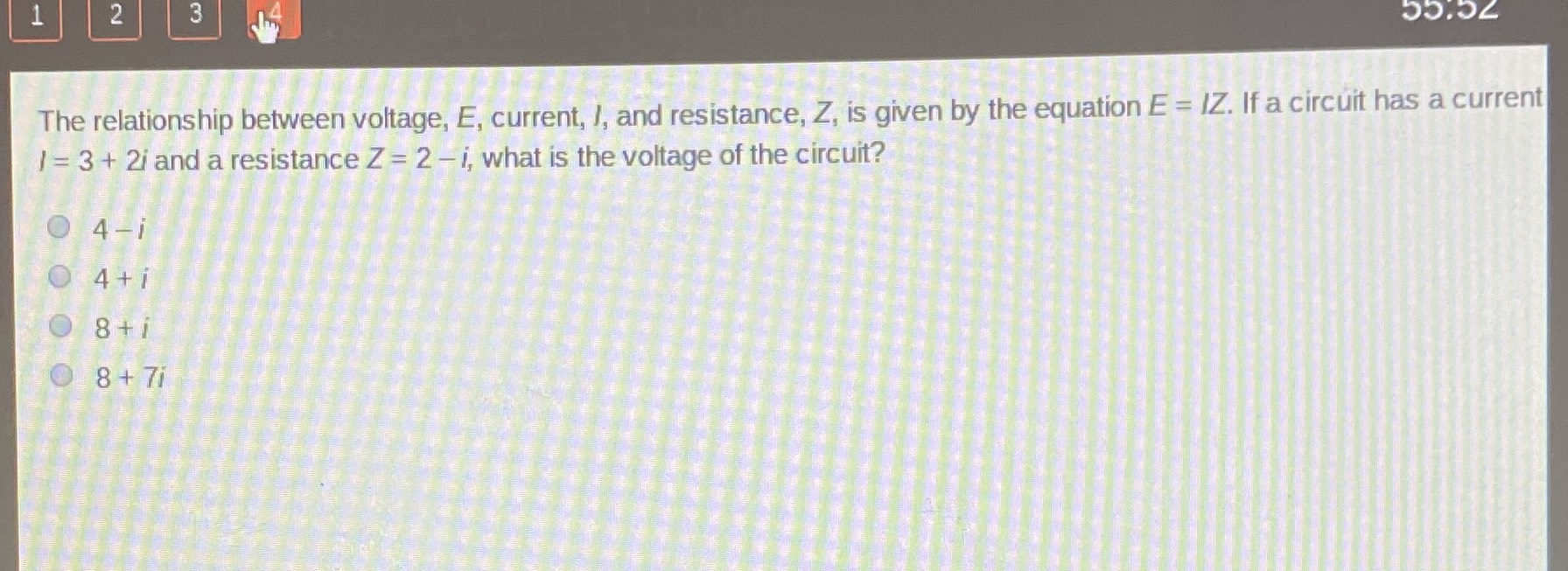### ¿Todavía tienes preguntas de matemáticas?

Pregunte a nuestros tutores expertos
Algebra
Pregunta$$142$$ The relationship between voltage, $$E$$ , current, $$l ,$$ and resistance, $$Z$$ , is given by the equation $$E = I Z$$ . If a circuit has a current $$I = 3 + 2 i$$ and a resistance $$Z = 2 - i ,$$ what is the voltage of the circuit?

$$4 - i$$

$$4 + i$$

$$8 + i$$

$$8 + 7 i$$